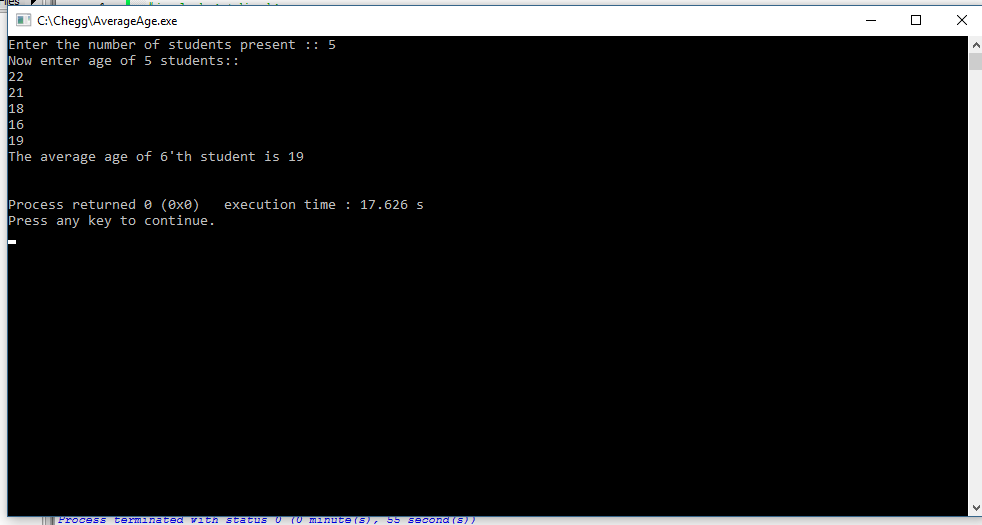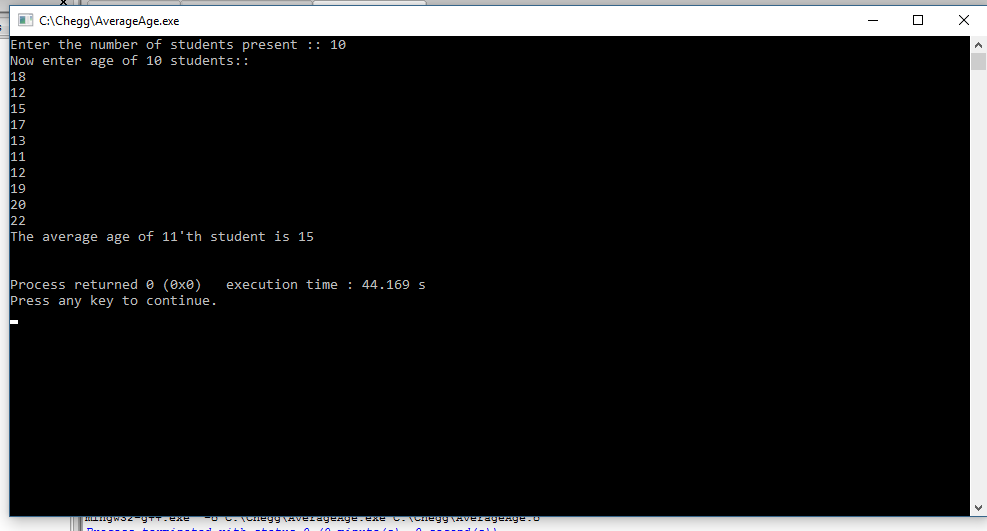# Question & Answer: Write a C program to compute the average age of nth student…..

Write a C program to compute the average age of nth student

Explanation::

Don't use plagiarized sources. Get Your Custom Essay on
Question & Answer: Write a C program to compute the average age of nth student…..
GET AN ESSAY WRITTEN FOR YOU FROM AS LOW AS \$13/PAGE
• Code in C language is given below.
• All the important syntax are explained in code itself in comments.
• Logi Applied::
• Step 1: Program ask user to enter the number of students age to entered. This value is stored in variable nin int datatype. Variable sum=0 of int type is also craeted to store total age of students.
• Step 2: An array named age[] of size n elements is created. It stores values in int datatype.
• Step 3: averageAge is calculated and printed accordingly.

Code in C language::

#include<stdio.h>
int main(){
/*
* Variable n stores how many students age is to be taken input.
* Variable sum is declared and initialize to sum=0. It stores sum of ages.
*/
int n,i,sum=0;
printf(“Enter the number of students present :: “);
scanf(“%d”,&n);
/*
* And Array named age of size n is created to store age of students in int data type.
* And in loop current age[i] is added to sum.
*/
int age[n];
printf(“Now enter age of %d students::n”,n);
for(i=0;i<n;i++){
scanf(“%d”,&age[i]);
sum=sum+age[i];
}
/*
* An variable named averageAge is declared and assigned average value of age.
* Average age is calculated in int data type
*
*/
int averageAge=sum/n;
int m=n+1; //to predict average of (n+1)’th student.
/*
* Finally average age is printed.
*/
printf(“The average age of %d’th student is %d”,m,averageAge);
printf(“nn”);
return 0;
}

Output::

Test Program Run 1::Test Program Run 2::